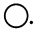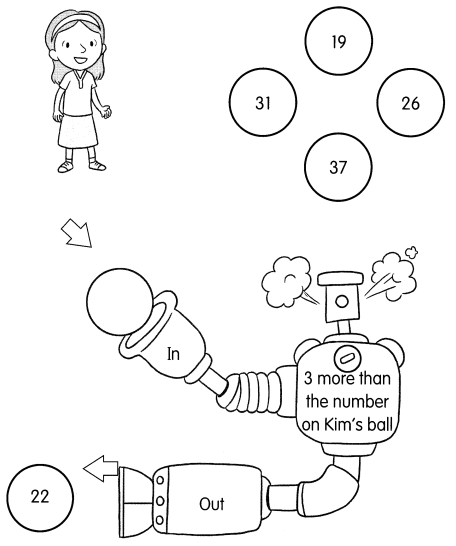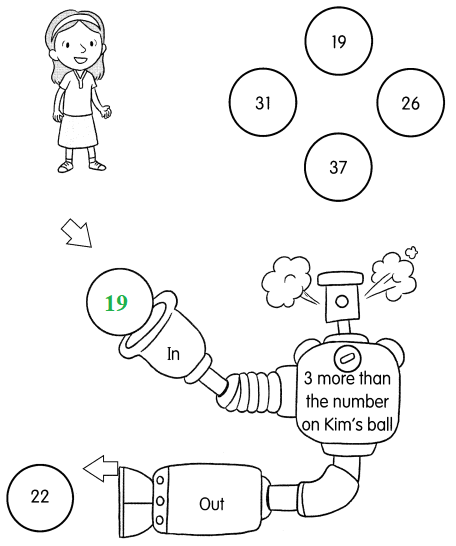# Math in Focus Grade 1 Chapter 12 Practice 3 Answer Key Comparing, Ordering, and Patterns

Practice the problems of Math in Focus Grade 1 Workbook Answer Key Chapter 12 Practice 3 Comparing, Ordering, and Patterns to score better marks in the exam.

## Math in Focus Grade 1 Chapter 12 Practice 3 Answer Key Comparing, Ordering, and Patterns

Find the missing numbers.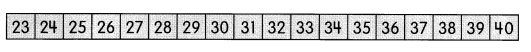Example

1 more than 23 is 24
1 less than 35 is 34

Question 1.
1 more than 29 is ___.
Answer: 1 more than 29 is 30

Question 2.
2 more than 19 is ____
Answer: 2 more than 19 is 21

Question 3.
2 more than 26 is ___.
Answer: 2 more than 26 is 28

Question 4.
2 less than 31 is ___.
Answer: 2 less than 31 is 29

Question 5.
3 more than 27 is ___.
Answer: 3 more than 27 is 30

Question 6.
3 more than 36 is ___.
Answer: 3 more than 36 is 39

Question 7.
3 less than 25 is ___.
Answer: 3 less than 25 is 22

Question 8.
3 less than 40 is ___.
Answer: 3 less than 40 is 37

Question 9.
____ is 2 more than 27.
Answer: 29 is 2 more than 27.

Question 10.
____ is 2 less than 26.
Answer: 28 is 2 less than 26.

Question 11.
____ is 3 more than 30.
Answer: 33 is 3 more than 30.

Question 12.
____ is 3 less than 30.
Answer: 27 is 3 less than 30.

Question 13.
___ is 2 more than 35.
Answer: 37 is 2 more than 35.

Question 14.
____ is 2 less than 35.
Answer: 33 is 2 less than 35.

Count the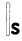in each set. Fill in the blanks.

Question 15.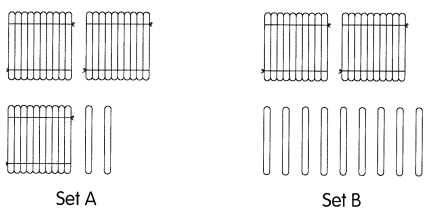Question
Which set has more? Set ____
Answer: Set A has more than set B
Question
Which number is greater?
___ is greater than ____
Answer: 32 is greater than 29

Question 16.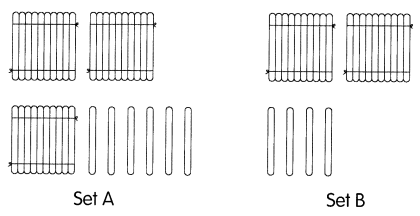Question
Which set has fewer? Set ____
Answer: Set A has fewer than Set b
Question
Which number is less?
____ is less than ____
Answer: 24 is less than 36

Circle the greater number.

Question 17.
32 or 23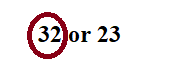Question 18.
37 or 39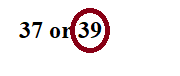Question 19.
19 or 21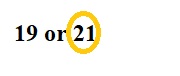Question 20.
15 or 25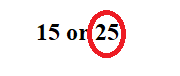Circle the number that is less.

Question 21.
32 or 28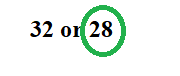Question 22.
38 or 40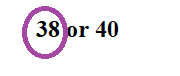Compare the numbers. Then fill in the blanks.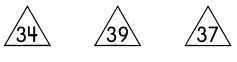Question 23.
____ is the least.

Question 24.
____ is the greatest.

Order the numbers from least to greatest.

Question 25.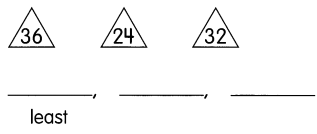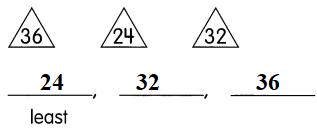Compare the numbers. Then fill in the blanks.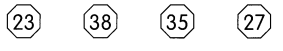Question 26.
________ is less than 27.
Answer: 23 is less than 27.

Question 27.
________ is greater than 35.
Answer: 38 is greater than 35.

Question 28.
35 is greater than ____ and ______ but less than ____.
Answer: 35 is greater than 27 and 23  but less than 38.

Question 29.
The least number is ______
Answer: The least number is 23

Question 30.
The greatest number is ____
Answer: The greatest number is 38

Compare the numbers. Then fill in the blanks.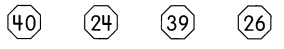Question 31.
________ is 2 more than 24.
Answer: 26 is 2 more than 24.

Question 32.
________ is 1 less than 40.
Answer: 39 is 1 less than 40.

Question 33.
________ is less than 39 but greater than 24.
Answer: 26 is less than 39 but greater than 24.

Question 34.
The least number is ____.
Answer: The least number is 24.

Question 35.
The greatest number is ________
Answer: The greatest number is 40

Complete each number pattern.

Question 36.
18, 19, ___, 21, ___, 23, _____.

Question 37.
30, 31, 32, ____, ____, 35, _____

Question 38.
____, ____, 30, 31, ___, 33, 34

Question 39.
33, ____, 31, ____, ____, 28, 27

Question 40.
30, 32, ___, ____, 38, ____

Question 41.
27, ___, ___, ___, 19, 17, 15

Question 42.
____, 23, 26, 29, ___, 35

Question 43.
33, ___, ___, 24, 21, ____
Kim’s ball falls into a number machine. Which ball is it? Write the missing number in the